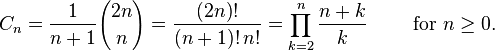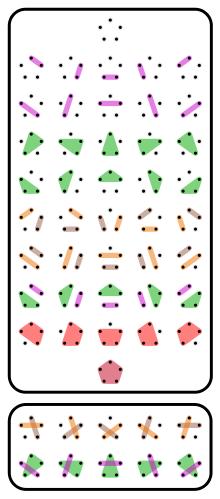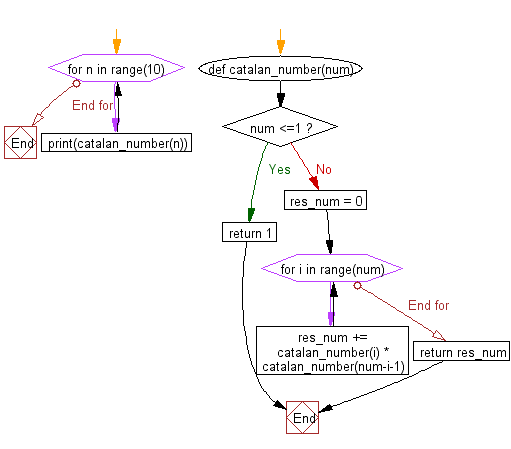﻿ Python Math: nth Catalan Number - w3resource# Python Math: nth Catalan Number

## Python Math: Exercise-25 with Solution

Write a Python program for nth Catalan Number.

In combinatorial mathematics, the Catalan numbers form a sequence of natural numbers that occur in various counting problems, often involving recursively-defined objects. They are named after the Belgian mathematician Eugène Charles Catalan (1814–1894).

Using zero-based numbering, the nth Catalan number is given directly in terms of binomial coefficients byThe first Catalan numbers for n = 0, 1, 2, 3, … are
1, 1, 2, 5, 14, 42, 132, 429, 1430, 4862, 16796, 58786, 208012, 742900, 2674440, ....

Pictorial Presentation

The C5 = 42 noncrossing partitions of a 5-element set (below, the other 10 of the 52 partitions)Image Credits: Watchduck

Sample Solution:-

Python Code:

``````def catalan_number(num):
if num <=1:
return 1

res_num = 0
for i in range(num):
res_num += catalan_number(i) * catalan_number(num-i-1)
return res_num

for n in range(10):
print(catalan_number(n))
```
```

Sample Output:

```1
1
2
5
14
42
132
429
1430
4862
```

Flowchart:## Visualize Python code execution:

The following tool visualize what the computer is doing step-by-step as it executes the said program:

Python Code Editor:

Have another way to solve this solution? Contribute your code (and comments) through Disqus.

What is the difficulty level of this exercise?

Test your Programming skills with w3resource's quiz.

﻿

```>>> students = [{'name': 'John', 'score': 98}, {'name': 'Mike', 'score': 94}, {'name': 'Jennifer', 'score': 99}]